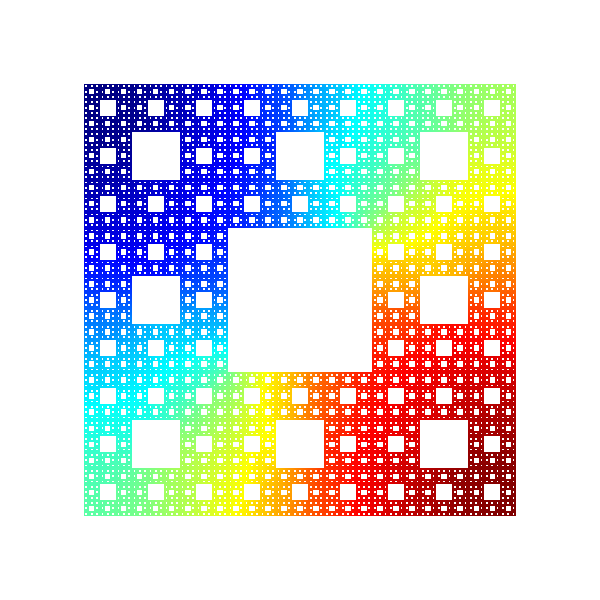# 6th Cornell Conference on Analysis, Probability, and Mathematical Physics on Fractals

June 13–17, 2017

## About Analysis and Probability on Fractals

Analysis and probability on fractals is an exciting new area of mathematical research that studies basic analytic operators and stochastic processes when the underlying space is fractal.  The following books give an indication of the accomplishments in this area in the recent past:

• Diffusions on Fractals by M. Barlow (Lecture Notes 1690, Springer, 1998),
• Analysis on Fractals by J. Kigami (Cambridge Univ. Press 2001),
• Differential Equations on Fractals: a tutorial (Princeton Univ. Press 2006) by R. Strichartz.

Research in this area is closely related to work in analysis and probability when the underlying space is manifold or a graph, and to analysis on metric measure spaces.

### Previous Meetings

Fractals 5 — June 11–15, 2014

Fractals 4 — September 10–13, 2011

Fractals 3 — June 11–15, 2008

Fractals 2 — May 31 – June 4, 2005